# Python training UGA 2017¶

A training to acquire strong basis in Python to use it efficiently

Pierre Augier (LEGI), Cyrille Bonamy (LEGI), Eric Maldonado (Irstea), Franck Thollard (ISTerre), Oliver Henriot (GRICAD), Christophe Picard (LJK), Loïc Huder (ISTerre)

# A short introduction to Matplotlib (gallery)¶

The default library to plot data is matplotlib. It allows one the creation of graphs that are ready for publications with the same functionality than Matlab.

In :
# these ipython commands load special backend for notebooks
# (do not use "notebook" outside jupyter)
# %matplotlib notebook
# for jupyter-lab:
# %matplotlib ipympl
%matplotlib inline


When running code using matplotlib, it is highly recommended to start ipython with the option --matplotlib (or to use the magic ipython command %matplotlib).

## Basic plotting with plot¶

In :
import numpy as np
import matplotlib.pyplot as plt

In :
y = np.random.random(5)
print(y)

[0.10778744 0.2393634  0.28269046 0.10192736 0.54835694]

In :
lines = plt.plot(y)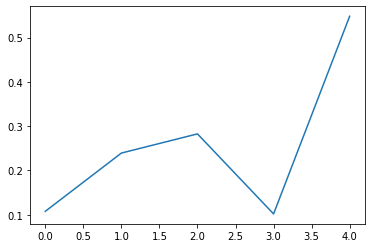In :
plt.show()


## Giving specific coordinates¶

In :
x = np.linspace(0, 2, 20)
y = x**2

plt.figure()
plt.plot(x, y)

Out:
[<matplotlib.lines.Line2D at 0x7fab75857910>]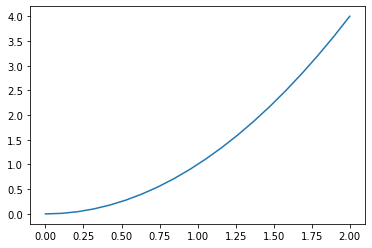## Figures and subplots¶

We can associate the figure and the system of axes to objects. These objects will allow us to add labels, modify the axis or save the figure.

The Figure object (in fig) handles the whole figure while the AxesSubplot object (in ax) handles the axes' system (called a subplot in matplotlib).

In :
fig, ax = plt.subplots()
# Old syntax:
# fig = plt.figure()
ax.plot(x, y)

# Example: Change x and y labels
ax.set_xlabel('$Re$')
ax.set_ylabel('$\Pi / \epsilon$')

Out:
Text(0, 0.5, '$\\Pi / \\epsilon$')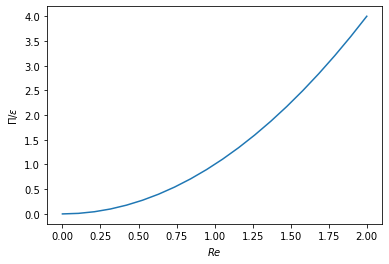### Multiple subplots¶

In :
fig, subplots = plt.subplots(nrows=2, ncols=1)
ax1, ax2 = subplots
X = np.arange(0, 2*np.pi, 0.1)
ax1.plot(X, np.cos(2*X), color="red")
ax2.plot(X, np.sin(2*X), color="magenta")

Out:
Text(0.5, 0, 'Angle (rad)')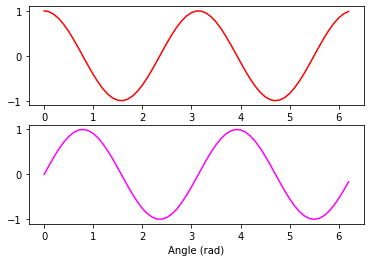### Customization options¶

There are a lot !

Matplotlib tries to make easy things easy and hard things possible.

See the documentation of plot for more details.

In :
fig, ax = plt.subplots()
res = ax.plot(x, y, color="red", linestyle='dashed', linewidth=3, marker='o',
markerfacecolor='blue', markersize=5)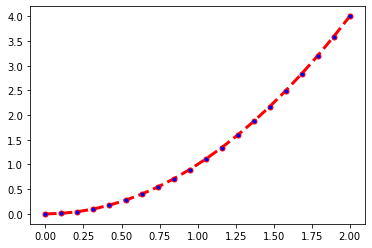We can also recover the plotted matplotlib object to get info on it.

In :
line_object = res
print(type(line_object))
print('Color of the line is', line_object.get_color())
print('X data of the plot:', line_object.get_xdata())

<class 'matplotlib.lines.Line2D'>
Color of the line is red
X data of the plot: [0.         0.10526316 0.21052632 0.31578947 0.42105263 0.52631579
0.63157895 0.73684211 0.84210526 0.94736842 1.05263158 1.15789474
1.26315789 1.36842105 1.47368421 1.57894737 1.68421053 1.78947368
1.89473684 2.        ]


### Titles, labels and legends¶

Titles can be added to figures and subplots. Labels can be added when plotting to generate a legend afterwards.

In :
x = np.arange(0, 2, 0.01)

fig, ax = plt.subplots()
ax.plot(x, x**2, label='$x^2$')
ax.plot(x, x**3, label='$x^3$')
ax.plot(x, np.exp(x) - 1, label='$e^{x} - 1$')
ax.set_title('Upscaling')
ax.legend()
fig.suptitle('FIGURE', fontweight='bold')

Out:
Text(0.5, 0.98, 'FIGURE')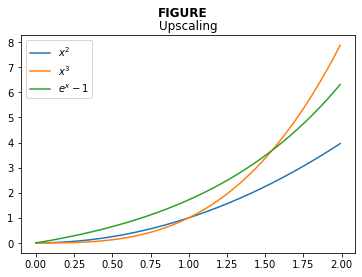Note that legends are attached to subplots. Note also the difference between the subplot title and the title of the figure.

## Anatomy of a Matplotlib figure¶For consistent figure changes, define your own stylesheets that are basically a list of parameters to tune the aspect of the figure elements. See https://matplotlib.org/tutorials/introductory/customizing.html for more info.

## 2D plots¶

There are two main methods:

• imshow: for square grids. X, Y are the center of pixels and (0,0) is top-left by default.
• pcolormesh (or pcolor): for non-regular rectangular grids. X, Y are the corners of pixels and (0,0) is bottom-left by default.
In :
noise = np.random.random((10,10))

f, subplots = plt.subplots(1, 2)
subplots.imshow(noise)
subplots.pcolormesh(noise)

Out:
<matplotlib.collections.QuadMesh at 0x7fab754f0cd0>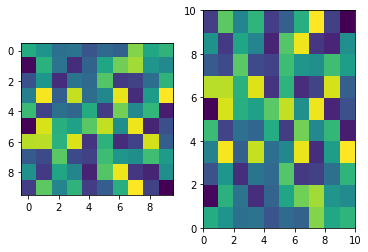In :
plt.figure()
plt.imshow(noise, cmap='Greys_r')
plt.colorbar()

Out:
<matplotlib.colorbar.Colorbar at 0x7fab754530d0>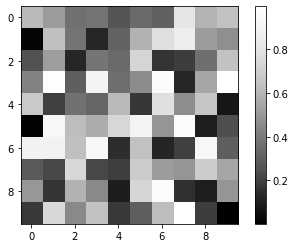### Generate meshes with meshgrid¶

When in need of plotting a 2D function, it is useful to use meshgrid that wil generate a 2D mesh from the values of abscissas and ordinates

In :
x = np.linspace(-2*np.pi, 2*np.pi, 200)
y = x

meshX, meshY = np.meshgrid(x, y)
Z = np.cos(2*meshX) + np.cos(4*meshY)

plt.figure()
plt.pcolormesh(meshX, meshY, Z, cmap='RdBu')
plt.colorbar()

Out:
<matplotlib.colorbar.Colorbar at 0x7fab753f2710>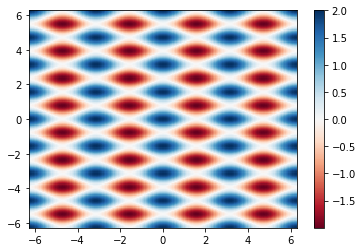### Choosing colormaps¶

When doing such colorplots, it is easy to lose the interesting features by setting a colormap that is not adapted to the data. As a rule of thumb:

• use sequential colormaps for data varying continously from a value to another (ex: x^2 for positive values)
• use divergent colormaps for data varying around a mean value (ex: cos(x))

Also, when producing scientific figures, think about how will your plot will look like to colorblind people or in greyscales (as it can happen in printed articles...).

See the interesting discussion on matplotlib website: https://matplotlib.org/users/colormaps.html.

In :
f, subplots = plt.subplots(1, 2)
plot1 = subplots.imshow(Z, cmap=plt.cm.jet)
f.colorbar(plot1, ax=subplots)
plot2 = subplots.imshow(Z, cmap=plt.cm.RdBu)
f.colorbar(plot2, ax=subplots)

Out:
<matplotlib.colorbar.Colorbar at 0x7fab7408bfd0>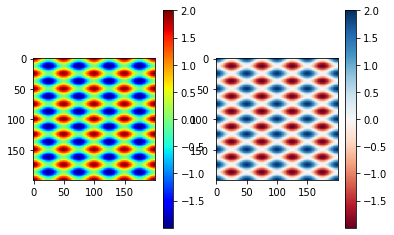## Other plot types¶

Matplotlib also allows to plot:

• Histograms
• Plots with error bars
• Box plots
• Contours
• in 3D
• ...

See the gallery to see what suits you the most.

In :
# 3D example
from mpl_toolkits.mplot3d import Axes3D

fig = plt.figure()

ax.plot_surface(meshX, meshY, np.exp(-(meshX**2+meshY**2)), cmap='viridis')

Out:
<mpl_toolkits.mplot3d.art3d.Poly3DCollection at 0x7fab6f7682d0>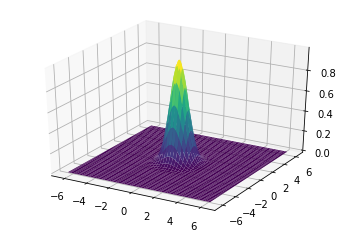## Do it yourself:¶

The pratical work below is mostly manipulation of an image (2D data). See TP5 for manipulation/plotting of 1D data.

With miscellaneous routines of scipy, we can get an example image:

In :
import scipy.misc
raccoon = np.array(scipy.misc.face())


Write a script to print shape and dtype the raccoon image. Next plot the image using matplotlib.

In :
print("shape of raccoon = ", raccoon.shape)
print("dtype of raccoon = ", raccoon.dtype)

shape of raccoon =  (768, 1024, 3)
dtype of raccoon =  uint8

In :
plt.imshow(raccoon)

Out:
<matplotlib.image.AxesImage at 0x7fab6eef98d0>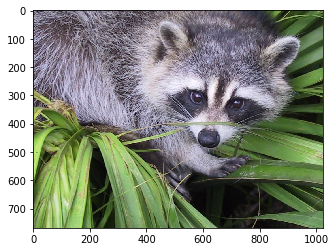1. Write a script to generate a border around the raccoon image (for example a 20 pixel size black border; black color code is 0 0 0)

2. Do it again without losing pixels and generate then a raccoon1 array/image

1. Mask the face of the raccoon with a grey circle (centered of radius 240 at location 690 260 of the raccoon1 image; grey color code is for example (120 120 120))
2. Mask the face of the raccon with a grey square by using NumPy broadcast capabilities (height and width 480 and same center as before)
3. We propose to smooth the image : the value of a pixel of the smoothed image is the the average of the values of its neighborhood (ie the 8 neighbors + itself).

### Solution 0¶

Write a script to generate a border around the raccoon image (for example a 20 pixel size black border; black color code is 0 0 0)

In :
raccoon[0:20, :, :] = 0
raccoon[-20:-1, :, :] = 0
raccoon[:, 0:20, :] = 0
raccoon[:, -20:-1, :] = 0
plt.imshow(raccoon)

Out:
<matplotlib.image.AxesImage at 0x7fab6ef34750>